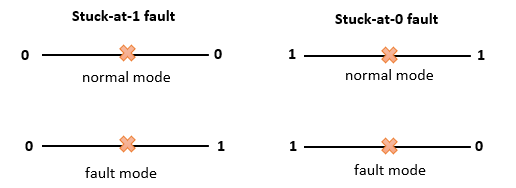## D-Algorithm -- Roth(1966)

The D-Algorithm (D-ALG) was developed by Roth in 1966.

Roth's D-Algorithm is a procedure that generates a specific circuit and a "fault" associated with that circuit which detects the absence or presence of a malfunction in the circuit.

A fault is any change of a circuit that affects the functioning of that circuit

In the D-Algorithm we describe one type of these faults in circuits, namely, the stuck-at faults.

In the D-Algorithm, we describe one type of fault in circuits: the stuck-at faults. Stuck-at-1 faults and stuck-at-0 faults can be described as pseudogates that have one input and one output.

In normal mode these pseudogates pass the proper signal through and in fault mode passes the negation signal.##### Overall purpose of Roth's D-Alorithm:

The overall purpose of this algorithm is that an integrated circuit and a fault can occur in the circuit. The algorithm locates a fault for this circuit, which detects the generated output.

That means, with a fault pseudogate, a given set of inputs detects the fault if the output differ from the expectet outputs.